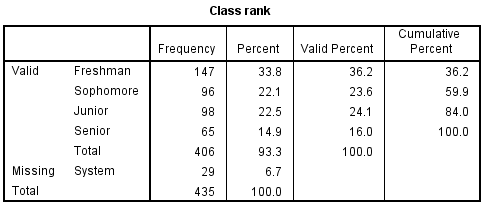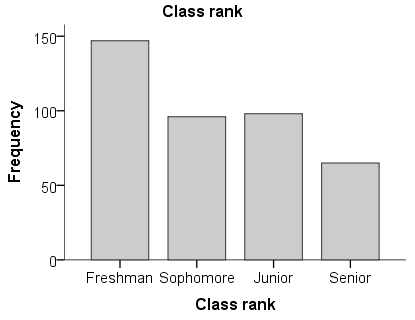# SPSS Tutorials: Frequency Tables

In SPSS, the Frequencies procedure is primarily used to create frequency tables, bar charts, and pie charts for categorical variables.

## Create a Frequency Table in SPSS

In SPSS, the Frequencies procedure can produce summary measures for categorical variables in the form of frequency tables, bar charts, or pie charts.

To run the Frequencies procedure, click Analyze > Descriptive Statistics > Frequencies.A Variable(s): The variables to produce Frequencies output for. To include a variable for analysis, double-click on its name to move it to the Variables box. Moving several variables to this box will create several frequency tables at once.

B Statistics: Opens the Frequencies: Statistics window, which contains various descriptive statistics.The vast majority of the descriptive statistics available in the Frequencies: Statistics window are never appropriate for nominal variables, and are rarely appropriate for ordinal variables in most situations. There are two exceptions to this:

• The Mode (which is the most frequent response) has a clear interpretation when applied to most nominal and ordinal categorical variables.
• The Values are group midpoints option can be applied to certain ordinal variables that have been coded in such a way that their value takes on the midpoint of a range. For example, this would be the case if you had measured subjects' ages and had coded anyone between the ages of 20 and 29 as 25, or between the 30 and 39 as 35 (source: IBM SPSS Statistics Information Center).

If your categorical variables are coded numerically, it is very easy to mis-use measures like the mean and standard deviation. SPSS will compute those statistics if they are requested, regardless of whether or not they are meaningful. It is up to the researcher to determine if these measures are appropriate for their data. In general, you should never use any of these statistics for dichotomous variables or nominal variables, and should only use these statistics with caution for ordinal variables.

C Charts: Opens the Frequencies: Charts window, which contains various graphical options. Options include bar charts, pie charts, and histograms. For categorical variables, bar charts and pie charts are appropriate. Histograms should only be used for continuous variables; they should not be used for ordinal variables, and should never be used with nominal variables.• Bar chart displays the categories on the graph's x-axis, and either the frequencies or the percentages on the y-axis
• Pie chart depicts the categories of a variable as "slices" of a circular "pie".

Note that the options in the Chart Values area apply only to bar charts and pie charts. In particular, these options affect whether the labeling for the pie slices or the y-axis of the bar chart uses counts or percentages. This setting will greyed out if Histograms is selected.

D Format: Opens the Frequencies: Format window, which contains options for how to sort and organize the table output.The Order by options affect only categorical variables:

• Ascending values arranges the rows of the frequency table in increasing order with respect to the category values: (alphabetically if string, or by numeric code if numeric)
• Descending values arranges the rows of the frequency table in decreasing order with respect to the category values.
• Note: If your categorical variable is coded numerically as 0, 1, 2, ..., sorting by ascending or descending value will arrange the rows with respect to the numeric code, not with respect to any assigned labels.)
• Ascending counts orders the rows of the frequency table from least frequent (lowest count) to most frequent (highest count).
• Descending counts orders the rows of the frequency table from most frequent (highest count) to least frequent (lowest count).

When working with two or more categorical variables, the Multiple Variables options only affects the order of the output. If Compare variables is selected, then the frequency tables for all of the variables will appear first, and all of the graphs for the variables will appear after. If Organize output by variables is selected, then the frequency table and graph for the first variable will appear together; then the frequency table and graph for the second variable will appear together; etc.

E Display frequency tables: When checked, frequency tables will be printed. (This box is checked by default.) If this check box is not checked, no frequency tables will be produced, and the only output will come from supplementary options from Statistics or Charts. For categorical variables, you will usually want to leave this box checked.

## What if my frequency table has a blank row in it?

What should I do if I create a frequency table in SPSS and one of the rows is blank?

If you are creating a frequency table using a string variable and notice that the first row has a blank category label, similar to this example:This particular issue is specific to frequency tables created from string variables. The blank row represents observations with missing values. SPSS does not automatically recognize blank (i.e., empty) strings as missing values, so the blank values appear as one of the "Valid" (i.e., non-missing) categories.

This issue should not be ignored! When missing values are treated as valid values, it causes the "Valid Percent" columns to be calculated incorrectly. If the blank values were correctly treated as missing values, the valid, non-missing sample size for this table would be 314 + 94 = 408 -- not 435! -- and the valid percent values would change to 314/408 = 76.9% and 94/408 = 23.0%. Depending on the number of missing values in your sample, the differences could be even more dramatic.

To fix this problem: To get SPSS to recognize blank strings as missing values, you'll need to run the variable through the Automatic Recode procedure. This procedure takes a string variable and converts it to a new, coded numeric variable with value labels attached. During this process, blank string values are recoded to a special missing value code. To see a worked example, see the Automatic Recode tutorial.

## Example: Summarizing a Categorical Variable

Using the sample dataset, let's a create a frequency table and a corresponding bar chart for the class rank (variable Rank), and let's also request the Mode statistic for this variable.

### Running the Procedure

#### Using the Frequencies Dialog Window

1. Open the Frequencies window (Analyze > Descriptive Statistics > Frequencies) and double-click on variable Rank.
2. To request the mode statistic, click Statistics. Check the box next to Mode, then click Continue.
3. To turn on the bar chart option, click Charts. Select the radio button for Bar Charts. Then click Continue.
4. When finished, click OK.

#### Using Syntax

FREQUENCIES VARIABLES=Rank
/STATISTICS=MODE
/BARCHART FREQ
/ORDER=ANALYSIS.

### Output

Two tables appear in the output: Statistics, which reports the number of missing and nonmissing observations in the dataset, plus any requested statistics; and the frequency table for variable Rank. The table title for the frequency table is determined by the variable's label (or the variable name, if a label is not assigned).Here, the Statistics table shows that there are 406 valid and 29 missing values. It also shows the Mode statistic: here, the mode value is "1", which is the numeric code for the category Freshman. Notice that the Mode statistic isn't displaying the value labels, even though they have been assigned. (For this reason, we recommend not requesting the mode statistic; instead, determine the mode from the frequency table.)Notice how the rows are grouped into "Valid" and "Missing" sections. This grouping allows for easy comparison of missing versus nonmissing observations. Note that "System" missing responses are observations that use SPSS's default symbol  -- a period (.) -- for indicating missing values. If a user has assigned special codes for missing values in the Variable View window, those codes would appear here.

The frequency table contains four columns of summary measures:

• The Frequency column indicates how many observations fell into the given category.
• The sample contained a total of 435 students. Of those students, 29 did not specify their class rank.
• The Percent column indicates the percentage of observations in that category out of all observations (both missing and nonmissing). You can verify the proportions for each group by dividing its count in the "frequency" column by the value in the last row of the table (435):
• Freshman: 147/435 = 33.8%
• Sophomore: 96/435 = 22.1%
• Junior: 98/435 = 22.5%
• Senior: 65/435 = 14.9%
• Valid Total: 406/435 = 93.3%
• Missing: 29/435 = 6.7%
• The Valid Percent column displays the percentage of observations in that category out of the total number of nonmissing responses. You can verify the proportions for each group by dividing its count in the "frequency" column by the value of "Total" that appears after the last valid category (406):
• Freshman: 147/406 = 36.2%
• Sophomore: 96/406 = 23.6%
• Junior: 98/406 = 24.1%
• Senior: 65/406 = 16.0%
• The Cumulative Percent column is the total percentage of the sample that has been accounted for up to that row; it can be computed by adding all of the numbers in the Valid Percent column above the current row:
• Freshman: 36.2% (there are no rows before this one, so the first cumulative percent is identical to the first valid percent)
• Sophomore: 36.2 + 23.6 = 59.8%
• Junior: 36.2 + 23.6 + 24.1 = 83.9%
• Senior: 36.2 + 23.6 + 24.1 + 16.0 = 100%

The bar chart appears after the tables.Here, we can see that:

• Freshmen comprised the largest group
• There were approximately equal numbers of sophomores and juniors
• Seniors were the smallest group